# C Program to Print the Address of a Variable

In this article, we will learn how to create a program in C that prints the address of any variable. At last, we will also learn about printing the address of a variable in C using a pointer.

```#include<stdio.h>
#include<conio.h>
int main()
{
int num;
printf("Enter any number to store in \"num\" variable: ");
scanf("%d", &num);
printf("\nValue of num = %d", num);
printf("\nAddress of num = %u", &num);
getch();
return 0;
}```

As the program was written in the Code::Blocks IDE, here is the sample run after a successful build and run: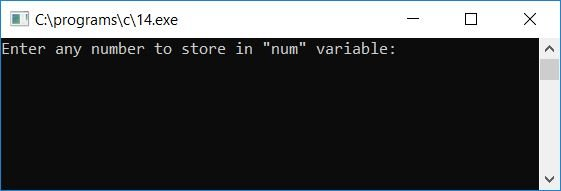Enter any number, say 10, and press the ENTER key to store it inside the variable num. You will see the value of the num variable along with its address: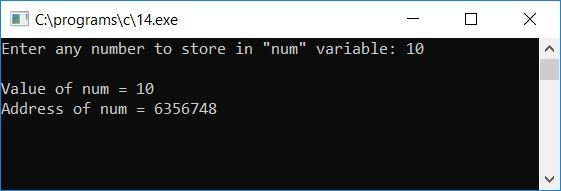#### Program Explained

• Receive any number from the user inside a variable, say num.
• Print back its value on the output screen.
• And to print the address of that variable, say num.
• We have to use the format specifier %u and add the address (&) operator before the variable, say num, so that &num will give the address of the num variable.

### Print the address of all variables

Now let's modify the above program. This program uses a total of 3 variables, say num1, num2, and num3, with one extra variable, say sum (which stores the summation result of the given three numbers). and see how the addresses of variables change one by one.

```#include<stdio.h>
#include<conio.h>
int main()
{
int num1, num2, num3, sum;
printf("Enter any number to store in \"num1\" variable: ");
scanf("%d", &num1);
printf("Enter any number to store in \"num2\" variable: ");
scanf("%d", &num2);
printf("Enter any number to store in \"num3\" variable: ");
scanf("%d", &num3);
printf("\nValue of num1 = %d", num1);
printf("\nAddress of num1 = %u", &num1);
printf("\nValue of num2 = %d", num2);
printf("\nAddress of num2 = %u", &num2);
printf("\nValue of num3 = %d", num3);
printf("\nAddress of num3 = %u", &num3);
sum = num1+num2+num3;
printf("\n\nValue of sum = %d", sum);
printf("\nAddress of sum = %u", &sum);
getch();
return 0;
}```

Here is its sample run: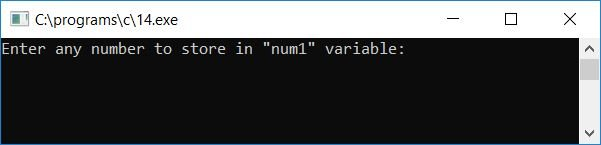Now enter any three numbers to store inside the variables num1, num2, and num3, respectively. And then press the ENTER key to see the values of all three variables, along with their addresses, on the output screenshot. We created another variable called sum to store the sum of all three variables, which we then printed on the output screen as its value and address: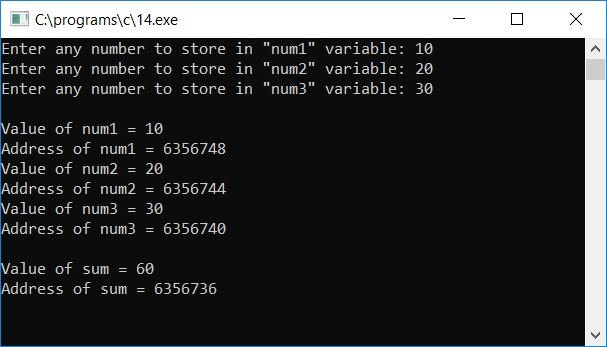### Print the value and address of each variable in the array

The question is: write a program in C to print the value and address of all the variables in an array. The answer to this question is:

```#include<stdio.h>
#include<conio.h>
int main()
{
int arr, max, i;
printf("How many elements you want to store in array: ");
scanf("%d", &max);
printf("Enter %d array elements: ", max);
for(i=0; i<max; i++)
scanf("%d", &arr[i]);
printf("\n");
for(i=0; i<max; i++)
{
printf("Value of arr[%d] = %d \t Address of arr[%d] = %u",i,arr[i],i,&arr[i]);
printf("\n");
}
getch();
return 0;
}```

Here is a sample run of the above program: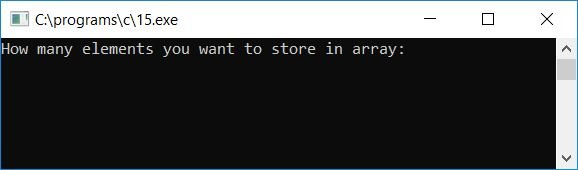Now enter any number, say 6, as the total number of elements that are going to be stored inside the array arr[]. Then enter all 6 array elements and press the ENTER key to see the following output: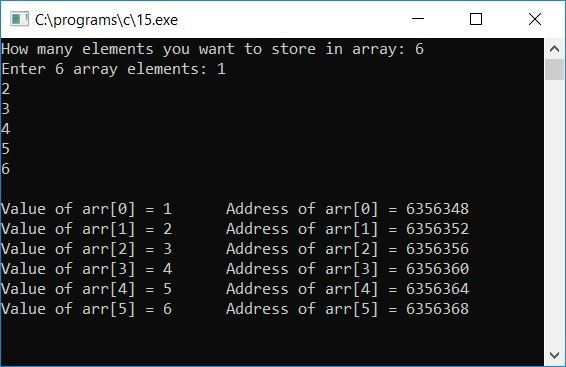### Print the value and address of variables in tubular form

Here is the modified version of the above program. This program prints the data (value and its address) in tubular form:

```#include<stdio.h>
#include<conio.h>
int main()
{
int arr, max, i;
printf("How many elements you want to store in array: ");
scanf("%d", &max);
printf("Enter %d array elements: ", max);
for(i=0; i<max; i++)
scanf("%d", &arr[i]);
for(i=0; i<max; i++)
{
printf("arr[%d]\t\t%d\t\t%u",i,arr[i],&arr[i]);
printf("\n");
}
getch();
return 0;
}```

Here is its sample run:## Pointer is used to print the address of a variable

Now let's create another program in C that prints the address of any variable using pointers:

```#include<stdio.h>
#include<conio.h>
int main()
{
int num1, *ptr1, num2, *ptr2;
printf("Enter the value of num1: ");
scanf("%d", &num1);
ptr1 = &num1;
printf("Address of num1 = %u", &ptr1);
ptr2 = &num2;
printf("\n\nEnter the value of num2: ");
scanf("%d", &num2);
ptr2 = &num2;
printf("Address of num2 = %u", &ptr2);
getch();
return 0;
}```

This is the first snapshot of the sample run: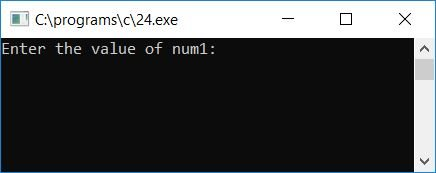Supply any number for the num1 variable and press the ENTER key to see the address of this variable, as shown in the screenshot given below: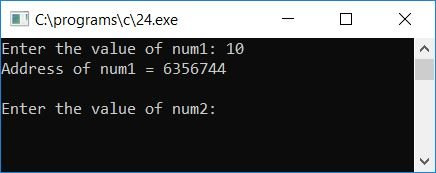Now again, supply any number for the num2 variable and press the ENTER key to see the address of the second variable as shown here: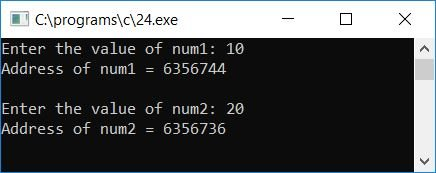#### Program Explained

• Declare any two-pointer integer variable.
• To declare any variable as a pointer variable, just place "*" before the variable.
• That is, *ptr1 and *ptr2 are two variables of pointer type.
• After removing the * before these two variables, both variables become normal variables.
• Now receive a number as input and store it inside the num1 variable.
• As a pointer variable is used to point to a variable's address, to initialize the address of any variable, just use &num1 to initialize address of the num1 variable to pointer.
• So, the ptr1 = &num1; statement says that the address of the num1 variable is set to the ptr1 pointer variable.
• Now, to print the value or address of num1, use the ptr1 pointer variable.
• To print address, use &ptr1, and to print value, use *ptr1. Here "&" is called the "address of" operator, and "*" is called the "value at" operator.
• Never forget to use the %u format specifier to print the address of any variable.

C Quiz

« Previous Program Next Program »